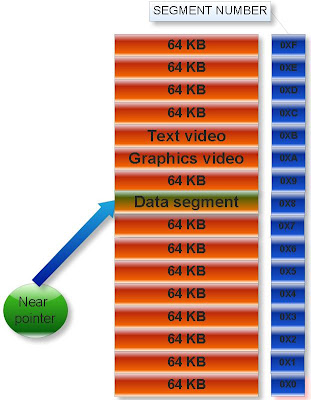## INDEX

### Near pointer in C programming

In TURBO C there are three types of pointers. TURBO C works under DOS operating system which is based on 8085 microprocessor.

1. Near pointer
2. Far pointer
3. Huge pointer

Near pointer:

The pointer which can points only 64KB data segment or segment number 8 is known as near pointer.That is near pointer cannot access beyond the data segment like graphics video memory, text video memory etc. Size of near pointer is two byte. With help keyword near, we can make any pointer as near pointer.

Examples:
(1)

#include<stdio.h>

int main(){

int x=25;
int near* ptr;

ptr=&x;
printf(“%d”,sizeof ptr);

return 0;
}

Output: 2

(2)

#include<stdio.h>

int main(){

int near* near * ptr;
printf(“%d”,sizeof(ptr),sizeof(*ptr));

return 0;
}

Output: 2 2
Explanation: Size of any type of near pointer is two byte.
Near pointer only hold 16 bit offset address. Offset address varies from 0000 to FFFF (in hexadecimal).

Note: In printf statement to print the offset address in hexadecimal, %p is used.

Example:

#include<stdio.h>

int main(){

int i=10;
int *ptr=&i;

printf("%p",ptr);

return 0;
}

%p is also used to print any number in hexadecimal number format.

Example:

#include<stdio.h>

int main(){

int a=12;
printf("%p",a);

return 0;
}

Output: 000C
Explanation: Hexadecimal value of 12 is C.

Consider the following two c program and analyze its output:

(1)

#include<stdio.h>

int main(){

int near * ptr=( int *)0XFFFF;
ptr++;
ptr++;
printf(“%p”,ptr);

return 0;
}

Output: 0003

(2)

#include<stdio.h>

int main(){

int i;
char near *ptr=(char *)0xFFFA;

for(i=0;i<=10;i++){
printf("%p \n",ptr);
ptr++;
}

return 0;
}

Output:

FFFA
FFFB
FFFC
FFFD
FFFE
FFFF
0000
0001
0002
0003
0004

Explanation: When we increment or decrement the offset address from maximum and minimum value respectively then it repeats the same value in cyclic order. This property is known as cyclic nature of offset address.

If you increment the near pointer variable then move clockwise direction. If you decrement the near pointer then move anti clockwise direction.What is default type of pointer in C?

Answer: It depends upon memory model.
What is memory model in C?

Generic pointer

Null pointer
Wild pointer
Dangling pointer
Near pointer
Far pointer
Graphics video memory
Text video memory
Huge pointer
Memory model in C
C tutorial